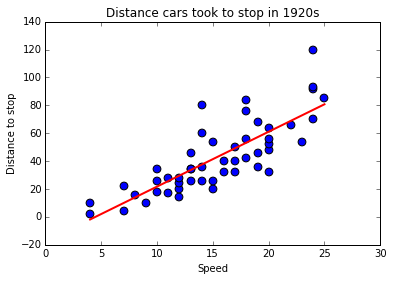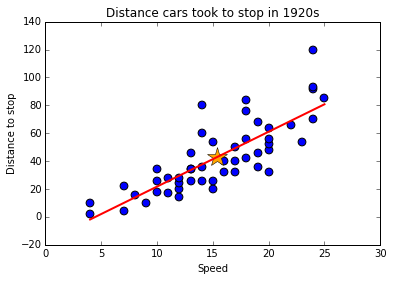In :
# import the packages required
import numpy as np
import scipy as sc
import statsmodels.api as sm
import matplotlib.pyplot as plt
# import the data set using statsmodel.api
cars_speed=sm.datasets.get_rdataset("cars", "datasets")


Let speed of the cars be X and distance they took to stop be Y.

In :
X=np.array(cars_speed.data['speed'])
Y=np.array(cars_speed.data['dist'])


Regression function $E\{Y_i\}=\beta_0+\beta_1X_i$ can be estimated as $\hat{Y}_i=b_0+b_1X_i$, where $b_0$ and $b_1$ are estimates for regression parameters $\beta_0$ and $\beta_1$ obtained using least squares. The formualas $b_0$ and $b_1$ are as follows:

\begin{equation} b_0=\bar{Y}-b_1\bar{X} \end{equation}\begin{equation} b_1=\frac{\sum (X_i-\bar{X})(Y_i-\bar{Y})}{\sum (X_i-\bar{X})^2} \end{equation}
In :
barX=np.mean(X); barY=np.mean(Y)
XminusbarX=X-barX; YminusbarY=Y-barY
b1=sum(XminusbarX*YminusbarY)/sum(XminusbarX**2)
b0=barY-b1*barX

In :
print 'b0=%4.5f' % b0, 'and', 'b1=%4.5f' % b1

b0=-17.57909 and b1=3.93241

In :
Yhat=b0+b1*X

In :
#make matplotlib inline
%matplotlib inline

#scatter plot
plt.scatter(cars_speed.data['speed'],cars_speed.data['dist'],c='b',s=60)

# xlable of the scatter plot
plt.xlabel('Speed')

# ylabel of the scatter plot
plt.ylabel('Distance to stop')

# title of the scatter plot
plt.title('Distance cars took to stop in 1920s')

# regression line
plt.plot(X,Yhat,'r-',linewidth=2)

Out:
[<matplotlib.lines.Line2D at 0x10c994f90>]Residuals are defined as $e_i=Y_i-\hat{Y}$

Properties of regression line:

1.$\sum e_i =0$

2.$\sum {e_i}^2$ is a minimum (requirement for least squares method).

3.$\sum{\hat{Y}_i} = \sum{Y_i}$

4.$\sum{X_ie_i}=0$

5.$\sum{\hat{Y}_ie_i}=0$

6.Regression line always goes through the point $(\bar{X},\bar{Y})$.

Next, let us test some of these properties for our example

In :
e_i=Y-Yhat # Residuals

In :
sum_of_residulas = np.sum(e_i)
sum_of_squares_of_residuals = np.sum(e_i**2)
print 'sum of residuals = %4.4f' % sum_of_residulas, ' ---property 1'
print 'sum of squares of residuals %4.4f' %sum_of_squares_of_residuals, '  ---property 2'
print 'sum of Y_i %4.4f' % np.sum(Y), '  ---property 3'
print 'sum of Yhat %4.4f' % np.sum(Yhat), '  ---property 3'
print 'sum of X_ie_i %4.4f' % np.sum(X*e_i), '  ---property 4'
print 'sum of Yhat e_i %4.4f' % np.sum(Yhat*e_i), '   ---property 5'

sum of residuals = 0.0000  ---property 1
sum of squares of residuals 11353.5211   ---property 2
sum of Y_i 2149.0000   ---property 3
sum of Yhat 2149.0000   ---property 3
sum of X_ie_i 0.0000   ---property 4
sum of Yhat e_i 0.0000    ---property 5

In :
# scatter plot
plt.scatter(cars_speed.data['speed'],cars_speed.data['dist'],c='b',s=60)
plt.xlabel('Speed')
plt.ylabel('Distance to stop')
plt.title('Distance cars took to stop in 1920s')

# point barX and barY
plt.plot(barX,barY,marker='*',markersize=20,color='orange')
#regression line
plt.plot(X,Yhat,'r-',linewidth=2)

print '  --- propert 6 (Orange point repersents the point xbar and ybar)'

  --- propert 6 (Orange point repersents the point xbar and ybar)Error sum of square or residual sum of square SSE is given by:

\begin{equation} SSE=\sum{{e_i}^2} \end{equation}

Error mean of square or residual mean of squares MSE is given by:

\begin{equation} MSE=\frac{\sum{{e_i}^2}}{n-2} \end{equation}

If $s^2$ is unbiased estimator of $\sigma^2$ then

$s^2=MSE$ and $s=\sqrt{MSE}$.

Also, $E\{MSE\}=\sigma^2$

In :
n=len(X); SSE=sum_of_squares_of_residuals; MSE=sum_of_squares_of_residuals/(n-2); s=np.sqrt(MSE)

In :
print  'SSE=%4.4f' % SSE, 'MSE=%4.4f' % MSE,'s=%4.4f' % s

SSE=11353.5211 MSE=236.5317 s=15.3796